Command Reference : Matrix Language Reference

 @covp
Pearson (population) product moment covariance (population – no d.f. adjustment).
(Same as @cov.)
Syntax: @covp(x)
x: matrix
Return: sym
Compute the covariance between columns of a matrix, with no d.f. correction.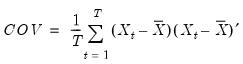where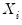is the column vector corresponding to the i-th row of the matrix,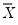are the corresponding means, and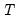is the number of rows of the matrix.
Examples
matrix x = @mnrnd(300, 5)
sym cov = @covp(@inner(x))
computes the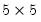non d.f. corrected covariance matrix of the randomly generated X.
Cross-references